# Heat Transfer in Power Resistors and the Use of Heat Sinks

Transferring heat energy generated by power resistors

This article deals with the practical matters of heat transfer in power resistors and includes a description of the specific geometry of film resistor parts, as opposed to other technologies such as wire wound resistors. When current is applied to a power film resistor (referred to as a "power resistor" in this article), the resistor element generates heat which must be dissipated in order that the temperature of the resistor element does not exceed its specified maximum operating temperature. Modern film power resistors are often incorporated into a wide variety of standard “TO” packages, which have the advantage of small size and convenient mounting in standard printed circuit board layouts. However, the small size of these standard packages will not support the power rating of these devices without additional help in transferring heat away from the resistor element. This help typically comes from attaching the package to a much larger thermal “heat sink”, which is in contact with a metal base plate or flange at the underside of the TO package. The purpose of the heat sink is to draw heat from the small resistor element (thus keeping the temperature of the element below its maximum operating temperature) and then dissipating the heat by conduction and convection over the much larger area of the heat sink.

Simple metal heat sinks

A simple, practical heat sink is an aluminum or steel plate as shown in Figure 1. When a resistor is attached directly to the heat sink, the heat generated by the resistor conducts to the metal plate, and then into the air as a result of convection. The cooling ability of the metal plate depends to a certain extent on whether the metal plate is oriented vertically or horizontally because of natural convective air cooling. Further heat transfer improvements can be obtained by forcing air over the heat sink in a process known as "forced-air cooling".

The heat transfer characteristics of heat sinks are determined experimentally and heat transfer values for aluminum and steel plates are well known. The cooling capacity of metal plate heat sinks in air are generally represented as “thermal resistance” (see next section), expressed as °C/W, of a metal-plate heat sink as shown in Figure 2. Figure 3 shows the thermal resistance of a more efficient heat sink incorporating fins.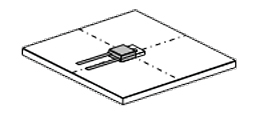Figure 1: Thermal source and metal plate in natural air cooling.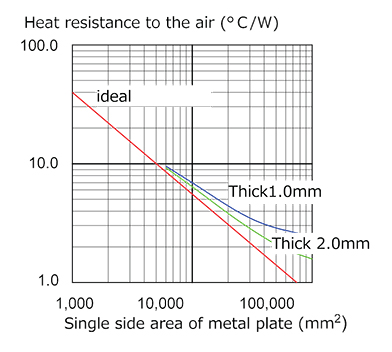Figure 2: Typical heat resistance of aluminum (measurements).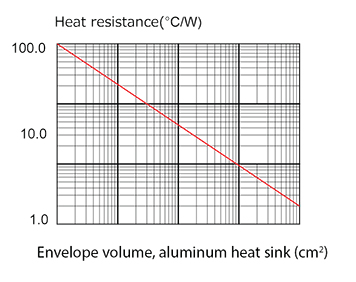Figure 3: Heat resistance against the air of aluminum cube with fin.

Thermal resistance, Rθ

Thermal resistance is a measure of resistance to the transfer of heat. In practice, thermal resistance is determined experimentally by measuring the temperature difference across the heat sink and dividing by the heat input in watts. Using the experimentally-determined thermal resistance of the device, the temperature difference between the entrance and exit of heat can be easily calculated from the heat (in watts) that passes through.

In Figure 2, the horizontal axis shows the area of an aluminum plate, while the vertical axis shows the thermal resistance of a natural air cooled aluminum plate when the heat source is mounted at the center of the plate. Thermal resistance makes the calculation easy. A resistor is placed in the center of a 0.1 mm thick aluminum plate with an area of 10,000 mm2 and an ambient temperature of 50°C. A power of 10 W is applied to the resistor. From Figure 2, the thermal resistance of the plate is about 7°C. Therefore, the difference between the temperature of the central portion and the ambient temperature will be 10 W x 7°C/W = 70°C and, if the air temperature is 50°C, the temperature of the central portion will be 50°C + 70°C = 120°C.

Internal thermal resistance of power resistors

The preceding section explained the process by which a heat sink transfers heat and represents the performance inherent to a heat sink.

In the same manner, a power resistor is structured so that the resistor itself generates heat internally and therefore the resistor itself has a specific thermal resistance. Figure 4 shows a cross-section of a power resistor.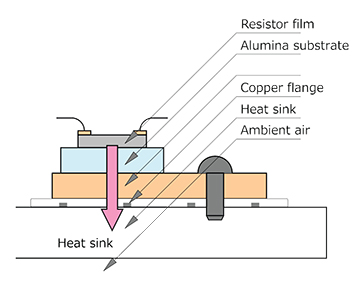Figure 4: Heat generation and thermal conduction.

 Riedon Part Resistor type Rated power Thermal resistance of resistor PF1262 TO126 20 W 5.9°C/W PF2202 TO220 20 W 5.9°C/W PF2203 TO220 35 W 3.3°C/W PF2205 TO220 50 W 2.3°C/W PF2472 TO247 100 W 1.3°C/W PF2272 SOT227 200 W 0.35°C/W

Table 1: Thermal resistances of power resistors.

As indicated in Table 1, the internal thermal resistances of power resistors are such that resistors with small outside dimensions and low-rated power levels have large thermal resistance values, while resistors with large dimensions and high-rated power have small values. Referring to the structure shown in Figure 4, it is not the outside dimensions but the area of the internal resistance film, the area of the alumina substrate and the area of the flange that determine the thermal resistance. In other words, the rated power is determined by the areas of the resistance film, alumina substrate, and flange.

The thermal grease shown in Figure 4 is not for electrically insulating the resistor flange but for filling the slight irregularities between the resistor flange surface and the heat sink surface to achieve better thermal conduction. Thermal grease is often used for this purpose. For a heat sink with a carefully finished surface, the thermal resistance of the thermal grease layer is around 0.005°C/W per 1 square inch, which is usually negligible as far as the cooling capacity is concerned.

As for heat conduction material between flange and heat sink as shown in Table 2, please note that the thickness of material is as important as thermal conductivity. Thermal grease is only about 50 microns thick while thermal gels and adhesives are around several hundred microns thick. The larger thickness increases the thermal resistance of the material and reduces cooling. Additionally, in power resistors there is no need to provide insulation between the back plate and heat sink, since they are already electrically insulated.

 Thermal conductivity (W/m × K) Recommendation Direct contact with good condition --- Yes Thermal conduction grease 3.2-8.0 Yes Thermal gel 6.5 No Thermal conduction adhesive 3.8 No

Table 2: Typical material recommendation between flange and heat sink

Example using thermal resistance to determine maximum power levels of TO-247

From the graph shown in Figure 2, the thermal resistance Rθ to air of an aluminum plate with a plate thickness of 2 mm and an expanded area of 40,000 mm2 is about 2.2°C/W. The thermal resistance of a TO247 resistor with a rated power of 100 W is 1.3°C/W and the flange contact thermal resistance is about 0.1°C/W. The upper limit on the operating temperature range of the resistance film of the power resistor is 155°C.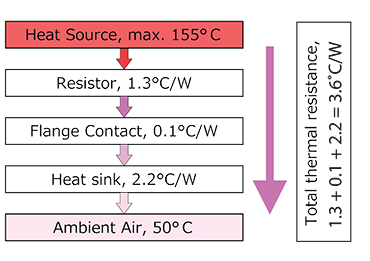Figure 5: Temperature at each portion in heat flow from resistor to air.

Thus, if the thermal resistance of the heat sink and the ambient temperature are determined, the maximum power which can be applied to the resistor without exceeding its maximum operating temperature can be determined. In the case of Figure 5, when the ambient temperature is 50°C, the maximum permissible power P = (155°C - 50°C) / 3.6 = 29 W. Notice that, if a resistor flange is mounted on a heat sink which is maintained at 25°C, say by forced air or liquid cooling, the maximum power which can be applied is also the rated power of the device = (155°C – 25°C) / 1.3 = 100 W. Generally, maximum power levels quoted in product specifications assume that the back plate (and therefore the heat sink at the contact area) is maintained at 25°C.

Figure 6 expresses a calculation of the thermal resistance above with the inclusion of a power derating curve. This is a calculated value. For actual devices, the important practical factors for reducing the random failure rate of the resistor are (1) accurately measuring the temperature distribution and (2) reducing the load by about 50% so that the resistor operates at a lower temperature.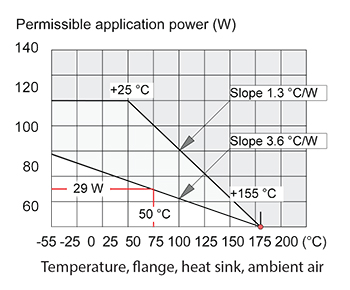Figure 6: Temperature at each portion in heat flow from resistor to air.

Mounting power resistors

Figures 7, 8, 9, 10, 11, 12 and 13 show examples of mounting with consideration given to heat release, together with rough values of thermal resistance.Figure 7: Thermal resistance to the ambient air of 35 μm single-sided copper foil paper phenol PCB of 100 mm square angles implementation vertically, 16.5°C/W.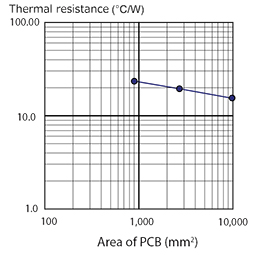Figure 8: Thermal resistance of PCB, 23.5°C/W (30 mm x 30 mm), 20°C/W (50 mm x 50 mm), 16.5°C/W (100 mm x 100mm).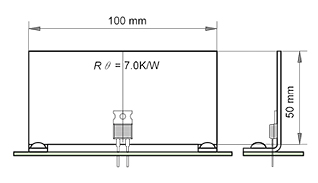Figure 9: Thermal resistance of 1 mm thickness aluminum plate heat sink with area 100 mm X 50 mm, 7°C/W.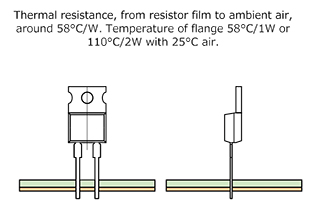Figure 10: Thermal resistance of the TO220 resistor without any heat sink.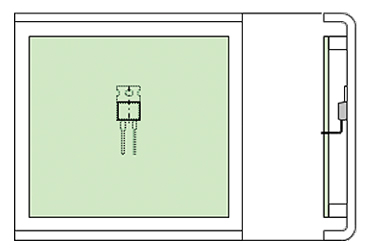Figure 11: Typical installation of power resistor (chassis mount).Figure 12: Typical installation of power resistor (board mount with standoff to chassis).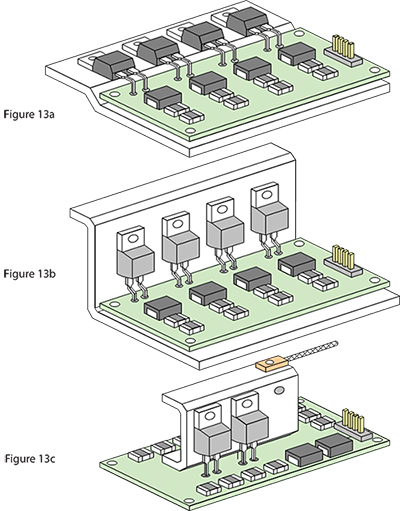Figures 13a, 13b, and 13c: Examples of mounting power resistors.

Riedon, Inc.